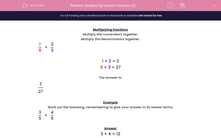# Multiplying Simple Fractions (2)

In this worksheet, students multiply simple pairs of proper fractions, writing the answer in its simplest form.This content is premium and exclusive to EdPlace subscribers.Key stage:  KS 2

Curriculum topic:   Maths and Numerical Reasoning

Curriculum subtopic:   Fractions

Difficulty level:#### Worksheet Overview

Multiplying fractions

Multiply the numerators together.

Multiply the denominators together.

 1 × 2 9 3

1 × 2 = 2

9 × 3 = 27

 2 27

Example

Work out the following, remembering to give your answer in its lowest terms.

 3 × 4 5 8

3 × 4 = 12

5 × 8 = 40

This gives:

 12 40

But this must be reduced by dividing top and bottom by 4.

 3 10

which we write as   3/10.

### What is EdPlace?

We're your National Curriculum aligned online education content provider helping each child succeed in English, maths and science from year 1 to GCSE. With an EdPlace account you’ll be able to track and measure progress, helping each child achieve their best. We build confidence and attainment by personalising each child’s learning at a level that suits them.

Get started